Hostname: page-component-546b4f848f-bvkm5 Total loading time: 0 Render date: 2023-05-31T04:31:54.869Z Has data issue: false Feature Flags: { "useRatesEcommerce": true } hasContentIssue false

# DEGREES OF CATEGORICITY AND SPECTRAL DIMENSION

Published online by Cambridge University Press:  01 May 2018

## Abstract

A Turing degree d is the degree of categoricity of a computable structure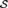${\cal S}$ if d is the least degree capable of computing isomorphisms among arbitrary computable copies of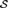${\cal S}$. A degree d is the strong degree of categoricity of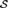${\cal S}$ if d is the degree of categoricity of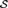${\cal S}$, and there are computable copies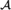${\cal A}$ and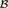${\cal B}$ of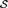${\cal S}$ such that every isomorphism from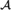${\cal A}$ onto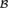${\cal B}$ computes d. In this paper, we build a c.e. degree d and a computable rigid structure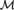${\cal M}$ such that d is the degree of categoricity of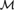${\cal M}$, but d is not the strong degree of categoricity of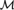${\cal M}$. This solves the open problem of Fokina, Kalimullin, and Miller .

For a computable structure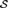${\cal S}$, we introduce the notion of the spectral dimension of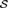${\cal S}$, which gives a quantitative characteristic of the degree of categoricity of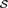${\cal S}$. We prove that for a nonzero natural number N, there is a computable rigid structure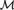${\cal M}$ such that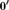$0\prime$ is the degree of categoricity of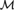${\cal M}$, and the spectral dimension of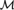${\cal M}$ is equal to N.

## Keywords

Type
Articles
Information
The Journal of Symbolic Logic , March 2018 , pp. 103 - 116

## Access options

Get access to the full version of this content by using one of the access options below. (Log in options will check for institutional or personal access. Content may require purchase if you do not have access.)

## References

#### REFERENCES

Anderson, B. A. and Csima, B. F., Degrees that are not degrees of categoricity. Notre Dame Journal of Formal Logic, vol. 57 (2016), no. 3, pp. 289398.Google ScholarPubMed
Ash, C. J., Recursive labelling systems and stability of recursive structures in hyperarithmetical degrees. Transactions of the American Mathematical Society, vol. 298 (1986), pp. 497514.CrossRefGoogle Scholar
Ash, C. J., Stability of recursive structures in arithmetical degrees. Annals of Pure and Applied Logic, vol. 32 (1986), pp. 113135.CrossRefGoogle Scholar
Ash, C. J. and Knight, J. F., Computable Structures and the Hyperarithmetical Hierarchy, Studies in Logic and the Foundations of Mathematics, vol. 144, Elsevier Science B.V., Amsterdam, 2000.Google Scholar
Bazhenov, N. A., Degrees of categoricity for superatomic Boolean algebras. Algebra Logic, vol. 52 (2013), no. 3, pp. 179187.CrossRefGoogle Scholar
Bazhenov, N. A.,${\rm{\Delta }}_2^0$-categoricity of Boolean algebras. Journal of Mathematical Sciences, vol. 203 (2014), no. 4, pp. 444454.CrossRefGoogle Scholar
Bazhenov, N. A., Autostability spectra for Boolean algebras. Algebra Logic, vol. 53 (2015), no. 6, pp. 502505.CrossRefGoogle Scholar
Bazhenov, N. A., Kalimullin, I. S., and Yamaleev, M. M., Degrees of categoricity vs. strong degrees of categoricity. Algebra Logic, vol. 55 (2016), no. 2, pp. 173177.Google Scholar
Csima, B. F., Franklin, J. N. Y., and Shore, R. A., Degrees of categoricity and the hyperarithmetic hierarchy. Notre Dame Journal of Formal Logic, vol. 54 (2013), no. 2, pp. 215231.CrossRefGoogle Scholar
Csima, B. F. and Stephenson, J., Finite computable dimension and degrees of categoricity, to appear. Available at www.math.uwaterloo.ca/∼csima/papers/finitecompdemdegcat.pdf.Google Scholar
Ershov, Y. L. and Goncharov, S. S., Constructive Models, Kluwer Academic/Plenum Publishers, New York, 2000.CrossRefGoogle Scholar
Fokina, E., Frolov, A., and Kalimullin, I., Categoricity spectra for rigid structures. Notre Dame Journal of Formal Logic, vol. 57 (2016), no. 1, 4557.CrossRefGoogle Scholar
Fokina, E. B., Kalimullin, I., and Miller, R., Degrees of categoricity of computable structures. Archive for Mathematical Logic, vol. 49 (2010), no. 1, pp. 5167.CrossRefGoogle Scholar
Frolov, A. N., Effective categoricity of computable linear orderings. Algebra Logic, vol. 54 (2015), no. 5, pp. 415417.CrossRefGoogle Scholar
Fröhlich, A. and Shepherdson, J. C., Effective procedures in field theory. Philosophical transactions of the Royal Society of London, Series A, vol. 248 (1956), 950, pp. 407432.CrossRefGoogle Scholar
Goncharov, S. S., Degrees of autostability relative to strong constructivizations. Proceedings of the Steklov Institute of Mathematics, vol. 274 (2011), pp. 105115.CrossRefGoogle Scholar
Hirschfeldt, D. R., Khoussainov, B., Shore, R. A., and Slinko, A. M., Degree spectra and computable dimensions in algebraic structures. Annals of Pure and Applied Logic, vol. 115 (2002), no. 1–3, pp. 71113.CrossRefGoogle Scholar
Mal’tsev, A. I., Constructive algebras. I. Russian Mathematical Surveys, vol. 16 (1961), no. 3, pp. 77129.CrossRefGoogle Scholar
Mal’tsev, A. I., On recursive abelian groups. Soviet Mathematics - Doklady, vol. 32 (1962), pp. 14311434.Google Scholar
Miller, R., d-computable categoricity for algebraic fields, this Journal, vol. 74 (2009), no. 4, pp. 1325–1351.Google Scholar
Miller, R. and Shlapentokh, A., Computable categoricity for algebraic fields with splitting algorithms. Transactions of the American Mathematical Society, vol. 367 (2015), no. 6, pp. 39553980.CrossRefGoogle Scholar